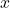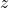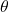# The ELBO without Jensen, Kullback, or Leibler

The log marginal likelihood is a central object for Bayesian inference with latent variable models:whereare observations,are latent variables, andare parameters. Variational inference tackles this problem by approximating the posterior overwith a simpler density. Often this density has a factored structure, for example. The approximating density is fit by maximizing a lower bound on the log marginal likelihood, or “evidence” (hence ELBO = evidence lower bound):The hope is that this will be a tight enough bound that we can use this as a proxy for the marginal likelihood when reasoning about. The ELBO is typically derived in one of two ways: via Jensen’s inequality or by writing down the KL divergence. Let’s quickly review these two derivations, and then I’ll show you a cute third derivation that doesn’t use Jensen’s inequality or K-L divergence.

### ELBO via Jensen’s Inequality

The Jensen’s approach observes that the expectation of a concave function is always less than or equal to that function evaluated at the expectation of its argument. That is, ifis concave, thenThere are two steps for the Jensen’s approach to the ELBO. First, multiply and divide inside the integral by, then apply Jensen’s inequality observing that the natural log is concave and that we now hav an expectation under:### ELBO via Kullback-Leibler Divergence

Alternatively, we could directly write down the KL divergence betweenand the posterior over latent variables,Now let’s both add and subtract the log marginal likelihoodfrom this:This log marginal likelihood doesn’t actually depend onso we can wrap it in an expectation underif we want. Let’s do that with the first one:Now turn the first two terms into a single expectation underand do the logarithm thing:Rearrange things slightly:We know that K-L divergences have to be non-negative so that shows us we have a lower bound on the log marginal likelihood.

### Alternative Derivationand observe that we can rearrange it to give us an expression for the marginal likelihood:This is true for any choice of. Siddhartha Chib has made some cool estimators using this trick, that I initially learned about from Iain Murray. Now let’s take the log of both sides and we’ll go on and stick the first two terms back together:Remember, this is true for all. Let’s add and subtract the log of an arbitrary density:Now, recall thatand so. ThereforeSince this is true for all, it’s also true in expectation under any distribution we want.is a natural choice:No reference here to Jensen’s inequality or K-L divergence. One caveat, however, is that the log inequality I used here is one way to prove non-negativity of K-L divergence. You could do this in a different order and it would look like directly taking advantage of the non-negativity of KL in the lower bound.